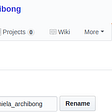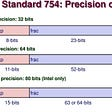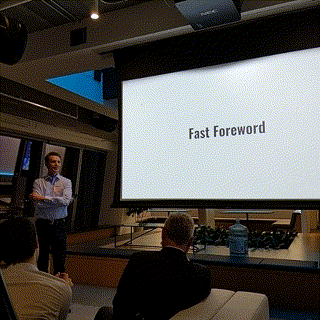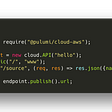# Tree Breadth First Search: Pattern for Coding Interviews

This pattern is based on the Breadth First Search (BFS) technique to traverse a tree or tree-like data structures.

Any problem involving the traversal of a tree in a level-by-level order can be efficiently solved using this approach. We will use a Queue to keep track of all the nodes of a level before we jump onto the next level.

Question asked at FAANG interviews: Binary Tree Level Order Traversal

Problem Statement : Given a binary tree, populate an array to represent its level-by-level traversal. You should populate the values of all nodes of each level from left to right in separate sub-arrays.

Hers’s an example below:

Try to brainstorm and implement the algorithm yourself before jumping to the solution below.

## Solution

Since we need to traverse all nodes of each level before moving onto the next level, we can use the Breadth First Search (BFS) technique to solve this problem.

We can use a Queue to efficiently traverse in BFS fashion. Here are the steps of our algorithm:

1. Start by pushing the root node to the queue.
2. Keep iterating until the queue is empty.
3. In each iteration, first count the elements in the queue (let’s call it levelSize). We will have these many nodes in the current level.
4. Next, remove levelSize nodes from the queue and push their values in an array to represent the current level.
5. After removing each node from the queue, insert both of its children(if exist) into the queue.
6. If the queue is not empty, repeat from step 3 for the next level.

Let’s take the example mentioned above to visually represent our algorithm:

Code(JavaScript)

`const Deque = require('./collections/deque'); class TreeNode {         // a typical tree node structureconstructor(val) {this.val = val;this.left = null;this.right = null;}}function bfsTraverse(root) {result = [];         // stores the final resultif (root === null) {return result;}const queue = new Deque();queue.push(root);   // add the root node initiallywhile (queue.length > 0) {const levelSize = queue.length;  currentLevel = [];for (i = 0; i < levelSize; i++) {currentNode = queue.shift();// add the node to the current levelcurrentLevel.push(currentNode.val);// insert the children of current node in the queueif (currentNode.left !== null) {queue.push(currentNode.left);}if (currentNode.right !== null) {queue.push(currentNode.right);}}result.push(currentLevel);}return result;}`

Time complexity of the above algorithm is O(N), where ’N’ is the total number of nodes in the tree. This is due to the fact that we traverse each node once.

Space complexity of the above algorithm will be O(N) as we need to return a list containing the level order traversal. We will also need O(N) space for the queue. Since we can have a maximum of N/2 nodes at any level (this could happen only at the lowest level), therefore we will need O(N) space to store them in the queue.

That’s all about Pattern — Breadth First Search. Will discuss more coding problems in the upcoming stories.

## More from Vishal Bhushan

Web Engineer

Love podcasts or audiobooks? Learn on the go with our new app.

## Blog Post_4## Bluehost Review: Prices, Ranking, Analysis, and Experience in 2020## Yes, This is my first blog entry stalker## How to deal with Floating-Point## Recap: Transit Techies #5: GTF5## Programming the Cloud## The Neglected Product Owner RoleWeb Engineer

## CS373 Spring 2022: Connor Popp Week 13## CS371p Spring 2022: Max Thomas## CS371p Spring 2022: Week 11## Prim’s Algorithm For Minimum Spanning Tree